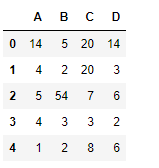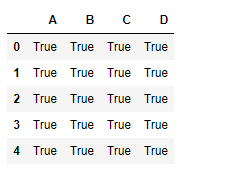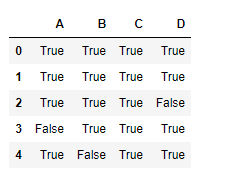# Python | Pandas dataframe.notna()

Python is a great language for doing data analysis, primarily because of the fantastic ecosystem of data-centric python packages. Pandas is one of those packages and makes importing and analyzing data much easier.

Pandas` dataframe.notna()` function detects existing/ non-missing values in the dataframe. The function returns a boolean object having the same size as that of the object on which it is applied, indicating whether each individual value is a `na` value or not. All of the non-missing values gets mapped to true and missing values get mapped to false.

Note : Characters such as empty strings ” or numpy.inf are not considered NA values. (unless you set pandas.options.mode.use_inf_as_na = True).

Syntax: DataFrame.notna()

Returns : Mask of bool values for each element in DataFrame that indicates whether an element is not an NA value

Example #1: Use `notna()` function to find all the non-missing value in the datafram.

 `# importing pandas as pd ` `import` `pandas as pd ` ` `  `# Creating the first dataframe  ` `df ``=` `pd.DataFrame({``"A"``:[``14``, ``4``, ``5``, ``4``, ``1``], ` `                   ``"B"``:[``5``, ``2``, ``54``, ``3``, ``2``],  ` `                   ``"C"``:[``20``, ``20``, ``7``, ``3``, ``8``], ` `                   ``"D"``:[``14``, ``3``, ``6``, ``2``, ``6``]}) ` ` `  `# Print the dataframe ` `df `Let’s use the `dataframe.notna()` function to find all the non-missing values in the dataframe.

 `# find non-na values ` `df.notna() `

Output :As we can see in the output, all the non-missing values in the dataframe has been mapped to true. There is no false value as there is no missing value in the dataframe.

Example #2: Use `notna()` function to find the non-missing values, when there are missing values in the dataframe.

 `# importing pandas as pd ` `import` `pandas as pd ` ` `  `# Creating the dataframe  ` `df ``=` `pd.DataFrame({``"A"``:[``12``, ``4``, ``5``, ``None``, ``1``], ` `                   ``"B"``:[``7``, ``2``, ``54``, ``3``, ``None``],  ` `                   ``"C"``:[``20``, ``16``, ``11``, ``3``, ``8``], ` `                   ``"D"``:[``14``, ``3``, ``None``, ``2``, ``6``]}) ` ` `  `# find non-missing values ` `df.notna() `

Output :As we can see in the output, cells which were having `na` values were mapped as false and all the cells which were having non-missing values were mapped as true.

My Personal Notes arrow_drop_upCheck out this Author's contributed articles.

If you like GeeksforGeeks and would like to contribute, you can also write an article using contribute.geeksforgeeks.org or mail your article to contribute@geeksforgeeks.org. See your article appearing on the GeeksforGeeks main page and help other Geeks.

Please Improve this article if you find anything incorrect by clicking on the "Improve Article" button below.

Article Tags :

1

Please write to us at contribute@geeksforgeeks.org to report any issue with the above content.